# Complex Numbers

A complex number is an expression of the form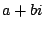, where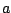and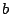are real numbers, and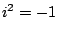. We add and multiply complex numbers as follows: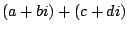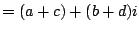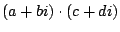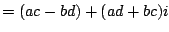The complex conjugate of a complex number is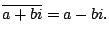Note that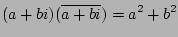is a real number (has no complex part).

If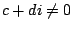, then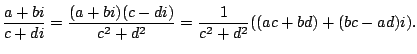Example 4.3.1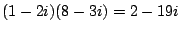and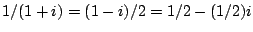.

Complex numbers are incredibly useful in providing better ways to understand ideas in calculus, and more generally in many applications (e.g., electrical engineering, quantum mechanics, fractals, etc.). For example,

• Every polynomial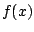factors as a product of linear factors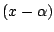, if we allow the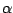's in the factorization to be complex numbers. For example,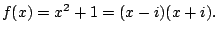This will provide an easier to use variant of the partial fractions'' integration technique, which we will see later.
• Complex numbers are in correspondence with points in the plane via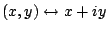. Via this correspondence we obtain a way to add and multiply points in the plane.
• Similarly, points in polar coordinates correspond to complex numbers: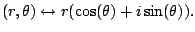• Complex numbers provide a very nice way to remember and understand trig identities.

Subsections
William Stein 2006-03-15